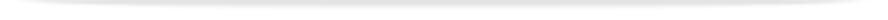units

ENG1090

Faculty of Engineeringprint version

This unit entry is for students who completed this unit in 2016 only. For students planning to study the unit, please refer to the unit indexes in the the current edition of the Handbook. If you have any queries contact the managing faculty for your course or area of study.

# ENG1090 - Foundation mathematics

## 6 points, SCA Band 2, 0.125 EFTSL

Refer to the specific census and withdrawal dates for the semester(s) in which this unit is offered.

Faculty

Engineering

Organisational Unit

Engineering

Coordinator(s)

Associate Professor Michael Page and Dr John Head

Offered

Clayton

• First semester 2016 (Day)

### Synopsis

Functions and coordinate geometry: types of functions, composite functions, inverse functions, modelling of periodic phenomena with trigonometric functions. Complex numbers. Differentiation and integration: concepts and techniques, applications to related rate of change and optimisation problems, areas, volume, and centre of mass. Vectors in two- and three-dimensional space, application to motion and kinematics.

### Outcomes

On successful completion of this unit, students will be able to:

1. Demonstrate understanding of the properties of common functions and their graphs, use composition of functions, and inverse functions; use trigonometric functions to model periodic behaviour.
2. Represent complex numbers in Cartesian, polar and exponential forms, and on the complex plane.
3. Perform arithmetic and algebra on complex numbers, including finding powers and complex roots of polynomials.
4. Demonstrate understanding of the concepts of limit, continuity, differentiable and integrable functions.
5. Evaluate limits of piecewise functions, and of rational functions at infinity.
6. Use differentiation rules to find derivatives of implicit and explicit functions.
7. Apply differentiation techniques to related rates of change problems and optimisation problems.
8. Use simple integration techniques to find definite and indefinite integrals, including by substitution and partial fractions.
9. Apply integration techniques to calculate areas, average values, volumes, centres of mass, moment, and work.
10. Perform operations with two and three-dimensional vectors, interpret them geometrically, calculate dot products, find vector resolutes, and apply them to motion of a particle.
11. Solve kinematics problems, and set up and solve problems involving Newton's laws of motion.
12. Express and explain mathematical techniques and arguments clearly in words.

### Assessment

Weekly Assignments or quizzes: 30%
Final examination (3 hours): 70%

Students are required to achieve at least 45% in the total continuous assessment component and at least 45% in the final examination component and an overall mark of 50% to achieve a pass grade in the unit. Students failing to achieve this requirement will be given a maximum of 45% in the unit.

Three 1 hour lectures ( or equivalent), one 2 hour practice class and 7 hours of private study per week